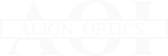Home    Products    Support    Company    Contact Us

The Precision People

# Simple Lens Approximations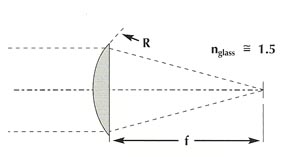For the plano-convex lens, the focal length equals twice the radius of the convex surface. This is also true for the plano-concave; howerver, since the radius is negative, this results in a negative focal length: f = 2R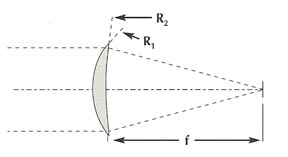For other forms of simple lenses, the following applies
 (Note: 0 = power): 1EFL = 0 = 12 [ 1R1 - 1R2 ]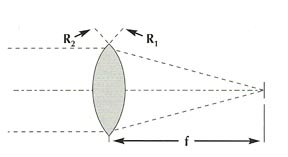For the double-convex or double-concave lens having equal radii, the focal length will equal the radius: R1 = R2 = f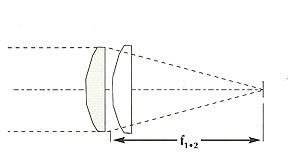If two or more thin lenses are stacked close together, their powers (1/EFL) may be added:
 0(1+2) = 01 = 02 = f(1+2) = f1 * f2f1 + f2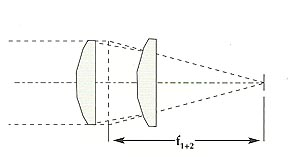If a substantial separation "d" exists between lenses, then:
 f(1+2) = f1 * f2f1 + f2 -d

 Optical Components Home | Site Map Our Networking Community | Optical Components Ploymer Optics © Align Optics - Designed by Doss Designs Align Optics 4700 Hiatus Rd., Ste. 143-A Sunrise, FL 33351 Phone: 954-748-1715 Toll Free 800-770-7320 Fax: 954-748-2149 E-Mail: sales@alignoptics.com